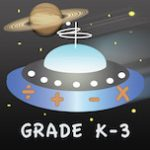@Reks: Educational iOS Applications

## 2nd Grade Printable Math Quiz Workshe...You can download a number of math practice worksheets designed for Grade 2  Elementary School. The practice worksheets are based on Math Essentials textbook and math common core standards. Unit 1 covers the following concepts: add and subtract numbers up to 20 add three or four numbers find partners and totals write equations for math mountains tell […]

## NEW App! Astro Math: Grades K-3Astro Math: Grades K-3 by @Reks is a reliable follow-up resource for common core standardized lessons. The app includes math facts covering kindergarten through 3rd grade school curriculum.